# Olympiad Test: Playing With Numbers - 1

## 10 Questions MCQ Test Mathematical Olympiad Class 8 | Olympiad Test: Playing With Numbers - 1

Description
Attempt Olympiad Test: Playing With Numbers - 1 | 10 questions in 20 minutes | Mock test for Class 8 preparation | Free important questions MCQ to study Mathematical Olympiad Class 8 for Class 8 Exam | Download free PDF with solutions
QUESTION: 1

### What is the next number in the series 5, 10, 26, 50, 122, ……

Solution:

5, 10, 26, 50, 122, …
(2)2 + 1, (3)+ 1, (5)2 + 1,
(7)2 + 1, (11)2 + 1, (13)2 + 1
∴ Next number = (13)2 + 1 = 170

QUESTION: 2

### A number is divisible by 63 if it is divisible by:

Solution:

63 = 7 × 9
∴ If a number is divisible by 63, then it should be divisible by both 7 and 9.

QUESTION: 3

### What is the sum of first 15 natural numbers?

Solution:

Sum of first ‘n’ natural numbers =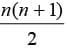∴ Sum of first 15 natural numbers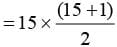= 15 × 8 = 120

QUESTION: 4

7,835 +  2b1 = 8126, then the value of b will be :

Solution: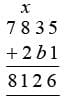Let ‘x’ be the carry, resulting from sum of b and 3.
8 + 2  + x = 11 ⇒ x = 1
∴ 3 + b = 12
⇒ b = 9

QUESTION: 5

What is the sum of first 20 odd numbers?

Solution:

Sum of ‘n’ odd natural numbers (first)= n2
∴ Sum of first 20 odd natural numbers = (20)2
= 400

QUESTION: 6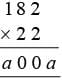, the value of ‘a’ will be :

Solution:

182 ×  22 = 4004
∴ a = 4

QUESTION: 7

What will be the last digit of 7333 ?

Solution:

71 = 7
72 = 49
7= 343
74 = 2401
75 = 16807

∴ After leaving 43 exponents 7n repeats its unit digit.
∴ 74n has 1 as its units place digit.
∴ 7332 has 1 as units place digit.
∴ 7333 has 7 as its units place digit.

QUESTION: 8

Which of the following numbers is divisible by 11?

Solution:

1221, 10·12-21 = 99, 99 can be divided by 11, hence 1221 is divisible by 11.

QUESTION: 9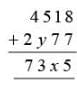the value of (x + y) will be :

Solution: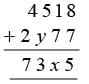When 8 and 7 are added, 1 is carry.
∴ x = 7 + 1 + 1 = 9
The carry of sum (5 + y) should be 1.
∴ 5 + y = 13
⇒ y = 8
∴ x + y = 17

QUESTION: 10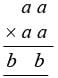, the value of (ab) will be : (1 ≤ a, b ≤ 9)

Solution: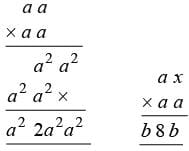⇒ a2 + a2 = 8
⇒ 2a2 = 8
⇒ a = 2, b = 4
∴ a × b = 8Use Code STAYHOME200 and get INR 200 additional OFF Use Coupon Code